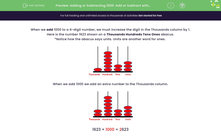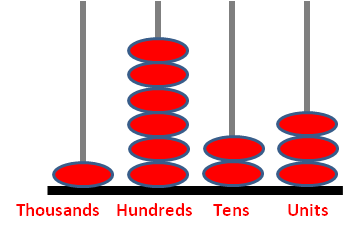### Comprehensive & curriculum aligned

In this worksheet, students must add 1000 or subtract 1000 from, a four-digit number.Key stage:  KS 2

Curriculum topic:   Number: Number and Place Value

Curriculum subtopic:   Find 1000 More or Less than a Given Number

Popular topics:   Subtraction worksheets, Addition worksheets

Difficulty level:#### Worksheet Overview

When we add 1000 to a 4-digit number, we must increase the digit in the Thousands column by 1.

Here is the number 1623 shown on a Thousands Hundreds Tens Ones abacus.

*Notice how the abacus says units. Units are another word for ones.When we add 1000 we add an extra number to the Thousands column.1623 + 1000 = 2623

Example

5028

We increase the 5 in the Thousands column by 1 to get 6 in that column.

5028 + 1000 = 6028

When we subtract 1000 from a 4-digit number, we must decrease the digit in the Thousands column by 1.

Here is the number 4705 shown on a Thousands Hundreds Tens Ones abacus.When we subtract 1000 from it, we take away one number from the Thousands column.4705 - 1000 = 3705

Example 1

Subtract 1000 from:

5097

We decrease the 5 in the Thousands column by 1 to get 4 in that column.

5097 - 1000 = 4097

Example 2

Subtract 1000 from:

1060

We decrease the 1 in the Thousands column by 1 to get 0 in that column.

Remember to leave off any zeros on the left hand side of a number.

1060 - 1000 = 0060 = 60

### What is EdPlace?

We're your National Curriculum aligned online education content provider helping each child succeed in English, maths and science from year 1 to GCSE. With an EdPlace account you’ll be able to track and measure progress, helping each child achieve their best. We build confidence and attainment by personalising each child’s learning at a level that suits them.

Get started